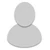## FANDOM

24,862 Pages

• So I know a it's a rule that no amount of Low 2-C multipliers will get you to 2-C, because the diatances between universes are unquantifiable. So what about multipliers for 2-C to 2-B or even 2-A? The diayance between universes is unquantifiable, but by making an explosion big enough to cover 2 universes, you already crossed said distance. We may not know how big the exolosion is, but we know it can cover inter-universal distances.

If we assume the distance between the second universe and a third universe is the same as the second and the first, then the explosion would logically need only to double in radius to cover 3 universes. We don't need it's exact size, we just need it to double what it originally was. It only takes around 8x the yield to go double the radius. I'm suggesting for this reason that multipliers be allowed in 2-C.

•